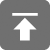# 工学1号馆

home

## C语言实现多态

Wu Yudong    June 27, 2015     C   575

1、巩固对面向对象机制的理解

2、巩固对C语言的理解

#include <stdio.h>
int main(){
printf("Hello World");
return 0;
}

ok，我们现在开始加快步伐

struct Square{
int width;
int height;
};

struct Circle{
};

struct Triangle{
int base;
int height;
};

printf("size of square is %d\n", sizeof(struct Square));
printf("size of Circle is %d\n", sizeof(struct Circle));
printf("size of Triangle is %d\n", sizeof(struct Triangle));

size of cube is 8

size of circle is 4

size of triangle is 8

void print_square( void ){
printf("Hello Square\n");
}

struct Square{
int width;
int height;
//now for functions
void (* print)( void );
float (* area)( struct Square * this );
};

print是一个指向参数为空、返回值为空的函数的指针，现在我们需要给Square一个面积函数，接受自身为参数，并且返回一个float类型来表示面积。读取的时候和print函数类似。

struct Square square;
square.print = print_square;

void init_square( struct Square * square, int w, int h ){
(*square).print = print_square;
(*square).width = w;
(*square).height = h;
(*square).area = calc_square_area;
}

#include<stdio.h>
struct Shape{
void (* print)( void );
float (* area)( struct Shape * this );
};

struct Square{
float width;
float height;
void (* print)( void );
float (* area)( struct Square * this );
};

void print_square( void ){
printf("Hello Square\n");
}

float calc_square_area(struct Square * this){
return (*this).width * (*this).height;
}

void init_square(struct Square * square, float w, float h ){
(*square).width = w;
(*square).height = h;
(*square).print = print_square;
(*square).area = calc_square_area;
}

int main(void)
{
struct Square square;
init_square( &square, 2.5, 2.6 );
square.print();
printf("the area of the square is %.2f\n", square.area(&square));
return 0;
}

//抽象父类
struct Shape{
void (* print)( void );
float (* area)( struct Shape * this );
};

struct Square square;
init_square(&square, 2, 4);

square.print();

struct Shape * pointer = (struct Shape *)&square;
(*pointer).print();    //?? 将会发生什么??

Square:

 width hight print area

4          4         4        4

Shape:

 print area

4        4

print()函数在Shape的内存模型中是前4个字节，在Square的内存模型是第三个4字节的位置

square.print();

(*pointer).print();

struct Shape{
void (* print)( void );
float (* area)( struct Shape * this );
};

#include<stdio.h>
//abstract father class
/*
struct Shape{
void (* print)( void );
float (* area)( struct Shape * this );
};
*/
struct Shape{
void (* print)( void );
float (* area)( struct Shape * this );
};

struct Square{
float width;
float height;

//now for functions
void (* print)( void );
float (* area)( struct Square * this );
};

void print_square( void ){
printf("Hello Square\n");
}

float calc_square_area(struct Square * this){
return (*this).width * (*this).height;
}

void init_square(struct Square * square, float w, float h ){
(*square).width = w;
(*square).height = h;
(*square).print = print_square;
(*square).area = calc_square_area;
}

int main(void)
{
struct Square square;
struct Shape *p = (struct Shape*)&square;
init_square( &square, 2, 2 );
//square.print();
(*p).print();
printf("the area of the square is %.2f\n", square.area(&square));
return 0;
}

BTW：这篇文章使用C来实现OOP的多态性质，不是最好的方法，仅仅是作为一种实现，后面将进一步优化改进

### 参考资料：

http://www.codeproject.com/Articles/739687/Achieving-polymorphism-in-C